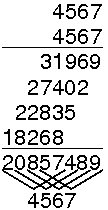# Hashing Tutorial

## Section 2.3 - Mid-Square Method

A good hash function to use with integer key values is the mid-square method. The mid-square method squares the key value, and then takes out the middle r bits of the result, giving a value in the range 0 to 2r-1. This works well because most or all bits of the key value contribute to the result. For example, consider records whose keys are 4-digit numbers in base 10. The goal is to hash these key values to a table of size 100 (i.e., a range of 0 to 99). This range is equivalent to two digits in base 10. That is, r = 2. If the input is the number 4567, squaring yields an 8-digit number, 20857489. The middle two digits of this result are 57. All digits of the original key value (equivalently, all bits when the number is viewed in binary) contribute to the middle two digits of the squared value. Thus, the result is not dominated by the distribution of the bottom digit or the top digit of the original key value. Of course, if the key values all tend to be small numbers, then their squares will only affect the low-order digits of the hash value. This image shows the long division process, and the relationship between the digits of the operator and the digits of the result.```
```

You should try out this hash function. Insert several values into the table. To simplify the presentation, the table size, key range, and bits multiplied and extracted are fixed. Look carefully at the results of the computation, and at which bits are being selected. Look at the effects on the resulting hash value from using both small numbers and larger numbers

The following applet will show you performance information about this hash function. Try with different table sizes, different levels of loading (the number of keys inserted), and different input distributions. In particular, see what happens when the hash table size is a power of 2 and an inappropriate key distribution is used, like an exponential distribution. In this applet, you may adjust how the Mid-square method does its calculation. Parameter P1 is the number of bits used in the calculation. Parameter P2 is the number of bits extracted from the middle of the P1 bits. For example, one could extract the middle 4 bits of 16 bits in the calculation, as was done in the applet above.